If you ever used Tableau, you know how easy and user-friendly it is for the end-user. Altair is a great Python library that allows you to program dashboard and other great stuff done in Tableau. Altair uses a so-called declarative approach in which we state what we want instead of stating how to get it.

# Before we start

We’ll be using Jupyter Lab on this one. First of all, install Jupyter Lab, Altaire and Vega :

pip install jupyterlab altair vega vega_datasets

Then, launch Jupyter Lab :

jupyter lab

We will use data from the French population that contains for each city :

• geolocation
• population
• population density

Just put the file in your working directory. We are now ready to go!

# The basics of Altair

Start by importing the Altair library and the file :

import altair as alt
france = 'france.csv'


If you want to further understand the structure of the data, read it using pandas :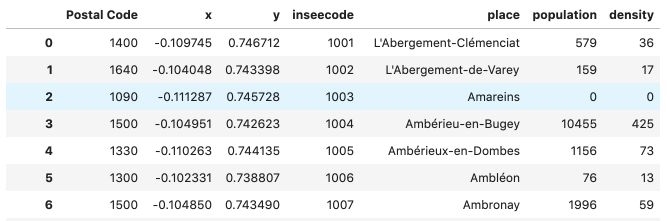## Basic principles

In Altair, to draw a Chart, you need to encode the variables to a certain type :

• Q: Stands for quantitative
• N: Nominal / Categorical data
• O: Ordinal data
• T: Temporal data

Let’s plot the map of France where the X-axis corresponds to the x column, and the Y-axis corresponds to the y column.

alt.Chart(france).mark_point().encode(
x='x:Q',
y='y:Q')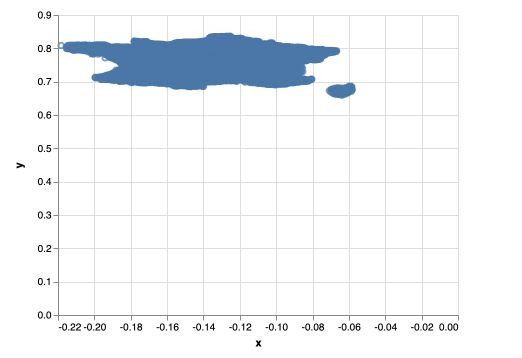• alt.Chart is used to declare the chart
• france is the path to our file
• mark_point is the type of marker we’re using. We’ll later see mark_circle or mark_square
• encode is used to encode the variables
• x='x:Q' means that we encode the column x to being quantitative. Same for the y column.

There is a major issue with the graph above, the origin of the plot is (0,0). We need to change that. We will also delete the axis values :

alt.Chart(france).mark_point().encode(
x=alt.X('x:Q', axis=None),
y=alt.Y('y:Q', axis=None, scale=alt.Scale(zero=False)))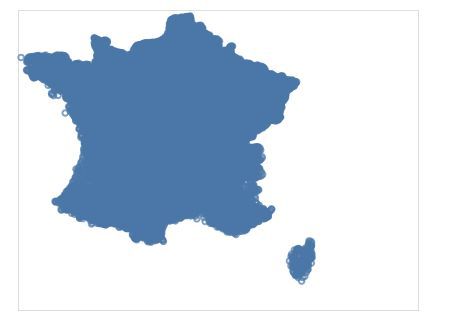Notice how we now declare the axis as objects in Altair using alt.X and alt.Y. We also disable the zero scale.

The points above are a bit too big. Let’s reduce their size in the mark_point. We will also assign the graph to a variable, and call that variable. We are also maxing the graph a bit bigger :

map = alt.Chart(france, width=600, height=600).mark_point(size=1).encode(
x=alt.X('x:Q', axis=None),
y=alt.Y('y:Q', axis=None, scale=alt.Scale(zero=False))
)

map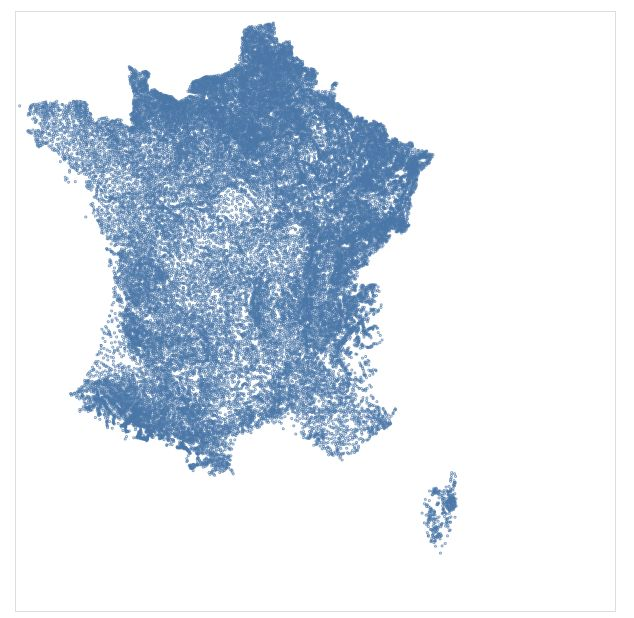## Size

Let’s now set the size of the marks accordingly to the population of the city.

map = alt.Chart(france, width=600, height=600).mark_point(size=1).encode(
x=alt.X('x:Q', axis=None),
y=alt.Y('y:Q', axis=None, scale=alt.Scale(zero=False)),
size='population:Q',
)

map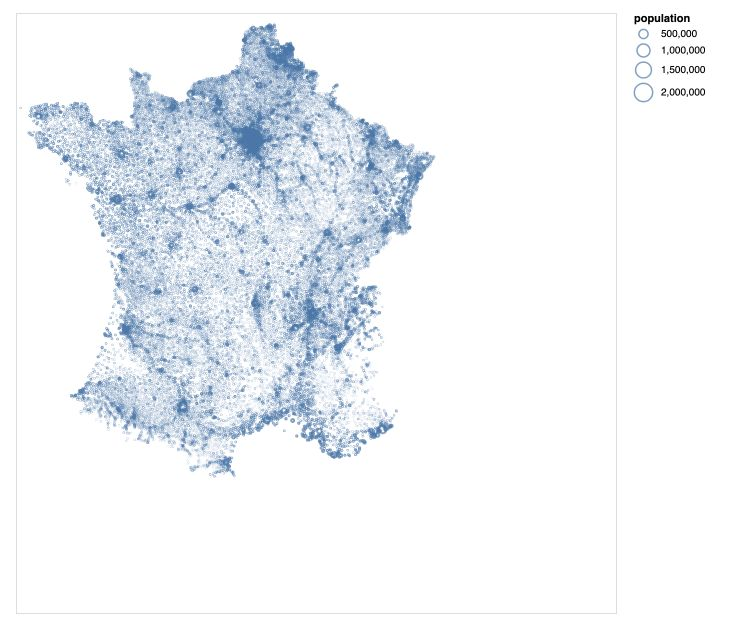Notice that we can also declare the size as an object itself using :

size = alt.Size('population:Q')


## Color

Remember that we do have the information of the population density per city. We can decide to set the color depending on the population’s density using alt.Color :

map = alt.Chart(france, width=600, height=600).mark_point(size=0.5).encode(
x=alt.X('x:Q', axis=None),
y=alt.Y('y:Q', axis=None, scale=alt.Scale(zero=False)),
size=alt.Size('population:Q'),
color=alt.Color('density:Q')
)

map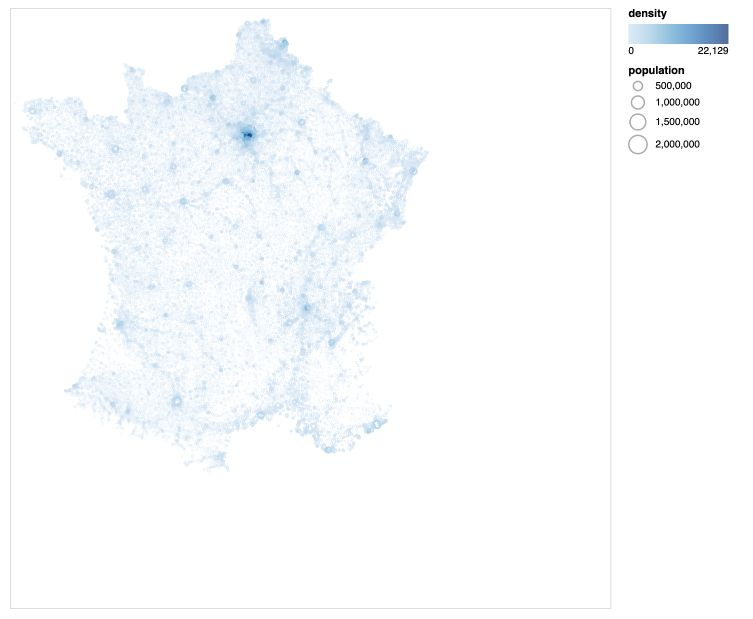It cool, but we have an issue here: the population’s density is extremely large in Paris and the Ile-de-France region, and the rest of France is too clear. Let’s now define a threshold (say a density of 2000) above which the color doesn’t change. We will also use a color template.

map = alt.Chart(france, width=600, height=600).mark_point(size=0.5).encode(
x=alt.X('x:Q', axis=None),
y=alt.Y('y:Q', axis=None, scale=alt.Scale(zero=False)),
size=alt.Size('population:Q'),
color=alt.Color('density:Q', scale=alt.Scale(scheme='viridis', domain=[0,2000]))

)

map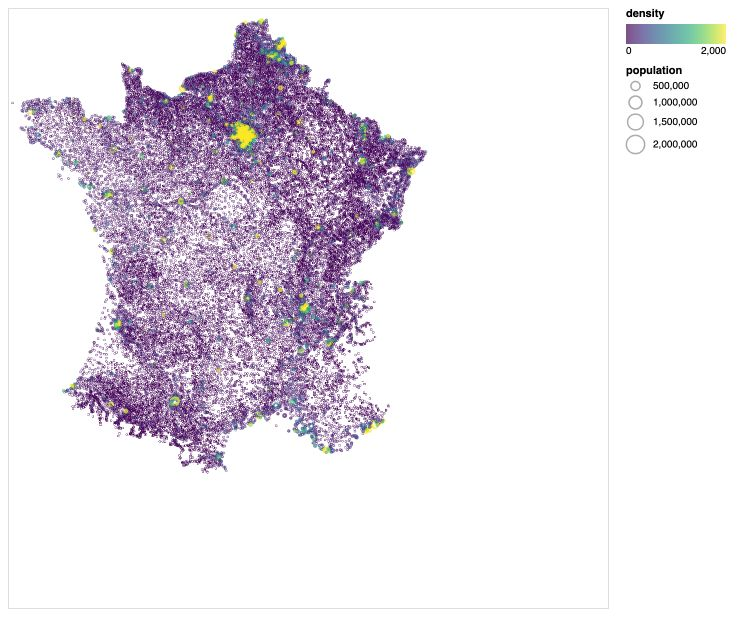That looks much better ! We can see the main cities of France appear in yellow on the map.

## Marks

So far, we’ve only used mark_point as a marker. We can specify the marker’s shape according to the following rules :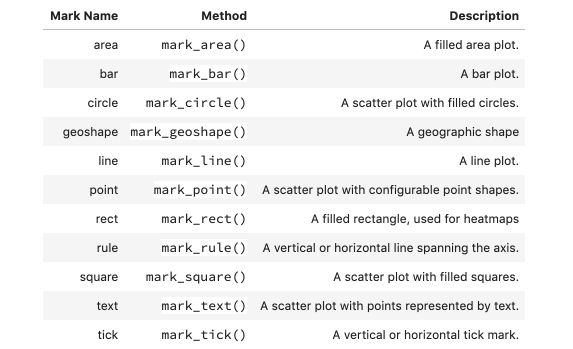For example :

map = alt.Chart(france, width=600, height=600).mark_square(size=1000).encode(
x=alt.X('x:Q', axis=None),
y=alt.Y('y:Q', axis=None, scale=alt.Scale(zero=False)),
size=alt.Size('population:Q'),
color=alt.Color('density:Q', scale=alt.Scale(scheme='viridis'))

)

map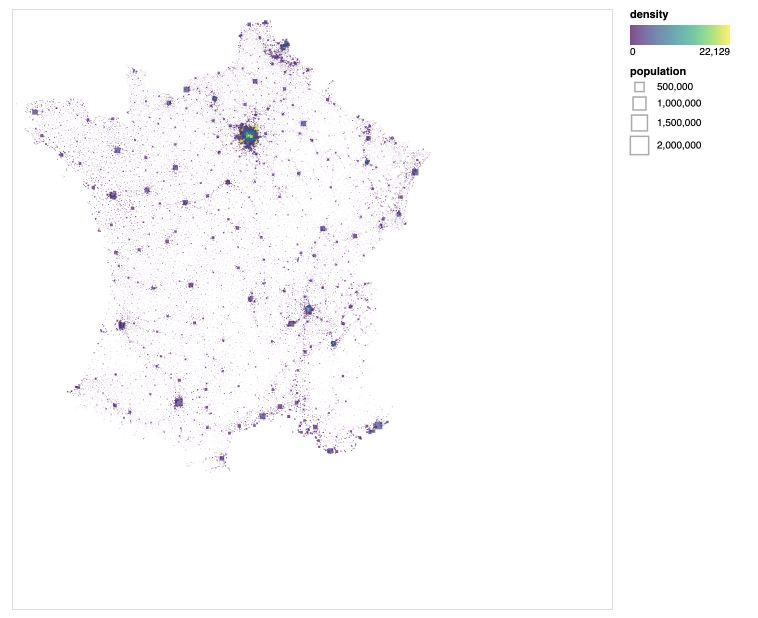## Histograms

To create histograms, there are three modifications to make :

• use mark_bar marker
• specify a bin, i.e the number of categories to create on the X-axis
• display the sum of the category (or the mean, count, std… for example) on the Y-axis
population = alt.Chart(france, width=600, height=300).mark_bar().encode(
x=alt.X('population:Q', bin=alt.Bin(maxbins=60)),
y='sum(population):Q')

population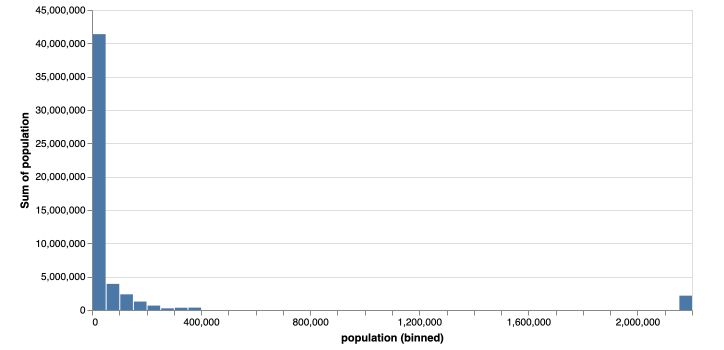Try to do the same for density on your side, and call it density.

## Heatmaps

If you add a bin on the Y-axis too, you obtain a heatmap! We will now create a heatmap of France, that aggregates information within the region (class X and class Y), and displays the count of the number of records within this region :

heat_map = alt.Chart(france, width=600, height=600).mark_square(size=50).encode(
x=alt.X('x:Q', axis=None, bin=alt.Bin(maxbins=90)),
y=alt.Y('y:Q', axis=None, scale=alt.Scale(zero=False), bin=alt.Bin(maxbins=90)),
color=alt.Color('count(population):Q', scale=alt.Scale(scheme='viridis')))

heat_map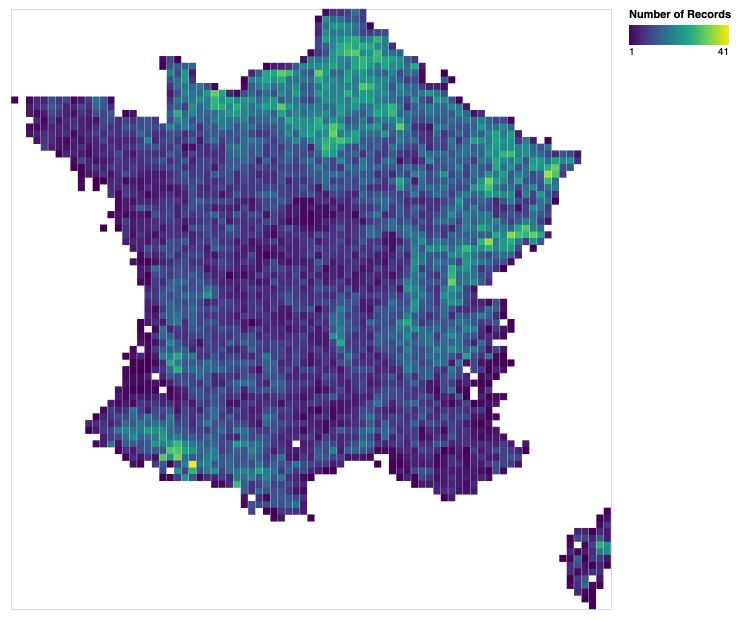## Dashboards

One of the nice features in Altair is to be able to build, as in Tableau, nice looking Dashboards. For example, here’s how to display the two histograms and the map of France we just built :

population.properties(height=100) & density.properties(height=100) & map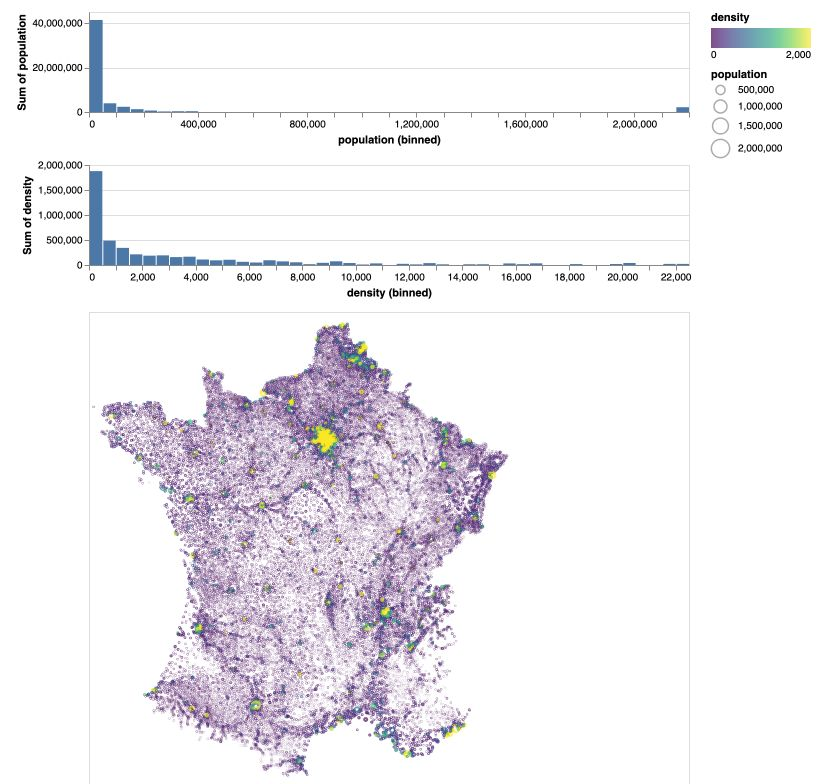# Interactive charts

Altair is great for developing interactive charts.

## Tooltips

The first step toward developing interactive charts is to integrate tooltips. Tooltips display a certain text message on overlay. For example :

map = alt.Chart(france, width=600, height=600).mark_point(size=0.5).encode(
x=alt.X('x:Q', axis=None),
y=alt.Y('y:Q', axis=None, scale=alt.Scale(zero=False)),
size=alt.Size('population:Q'),
tooltip=['place:N', 'population:Q', 'density:Q'],
color=alt.Color('density:Q', scale=alt.Scale(scheme='viridis', domain=[0,2000]))

)

map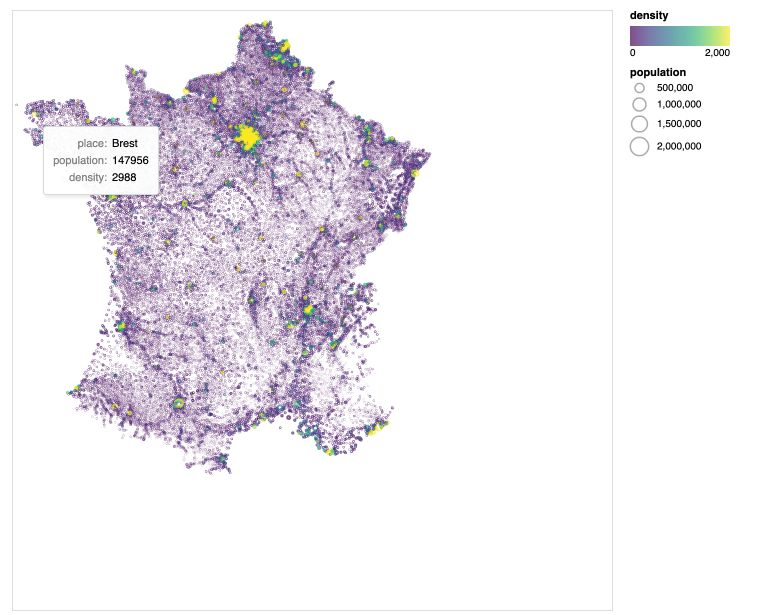## Selection intervals

In some cases, you might want to select a specific region of the map. This is done by adding a selection interval on the map :

brush = alt.selection_interval()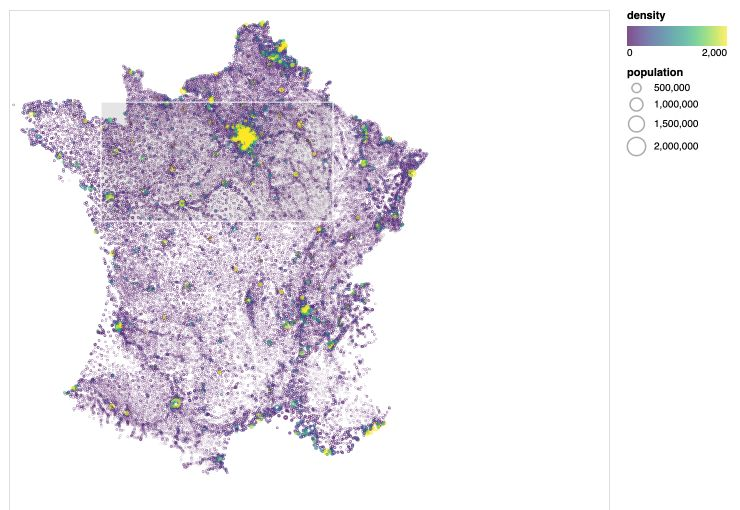As you might guess, this is quite pointless so far. We need to add more features, such as setting to gray the non-selected part using the alt.value argument in the color.

map = alt.Chart(france, width=600, height=600).mark_point(size=1).encode(
x=alt.X('x:Q', axis=None),
y=alt.Y('y:Q', axis=None, scale=alt.Scale(zero=False)),
size='population:Q',
tooltip=['place:N', 'population:Q', 'density:Q'],
color=alt.condition(brush, 'density:Q', alt.value('lightgrey'), scale=alt.Scale(scheme='viridis', domain=[0,2000]))

map


## Transform filters

At that point, this is a cool feature, but it remains useless. What we might want to do is on the Dashboard, when a region is selected on the map, update it on the histograms (to get for example the population’s histogram in this region).

This can be achieved using transform_filter.

population = population = alt.Chart(france, width=800, height=100).mark_bar().encode(
x=alt.X('population:Q', bin=alt.Bin(maxbins=60)),
y='sum(population):Q').transform_filter(brush)

population & map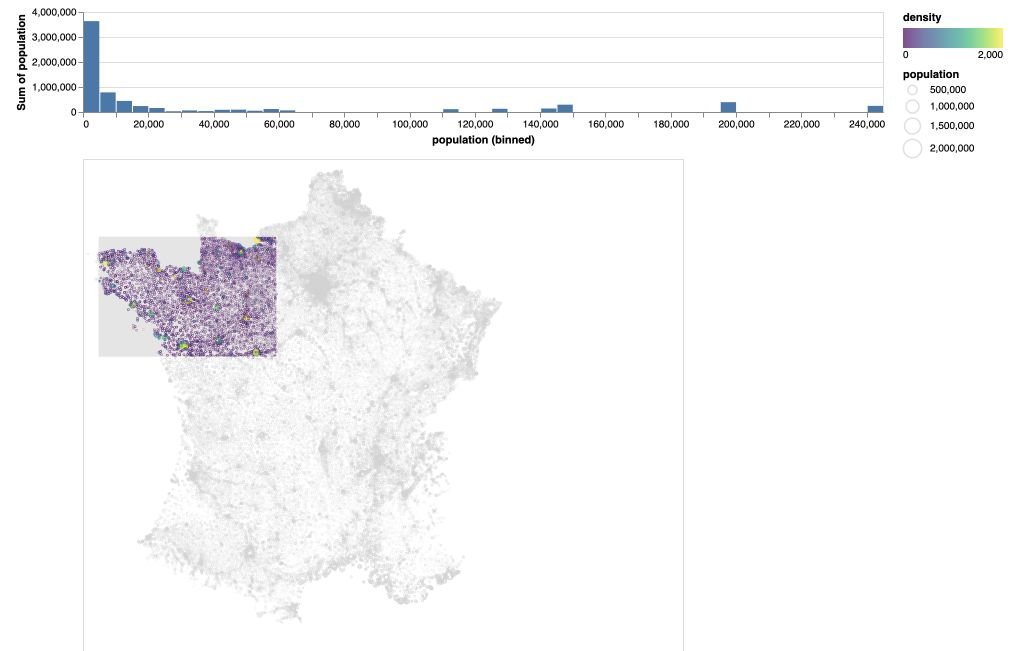As you can see, the transformation filter refers to the brush filter. This is interesting. The last improvement we’ll explore is to update the view in both ways. If we select a region of the histogram, it will update the map.

Start off by defining the two selection intervals :

brush = alt.selection_interval()
pop_selection = alt.selection_interval(encodings=['x'])


Then, define the map and add a selection according to population, and define the population histogram, add the population selection and add the transformation.

map = alt.Chart(france, width=600, height=600).mark_point(size=1).encode(
x=alt.X('x:Q', axis=None),
y=alt.Y('y:Q', axis=None, scale=alt.Scale(zero=False)),
size='population:Q',
color=alt.condition(pop_selection, 'density:Q', alt.value('lightgrey'), scale=alt.Scale(scheme='viridis', domain=[0,2000]))

population = alt.Chart(france, width=600, height=100).mark_bar().encode(
x=alt.X('population:Q', bin=alt.Bin(maxbins=50)),
y='sum(population):Q'

population & map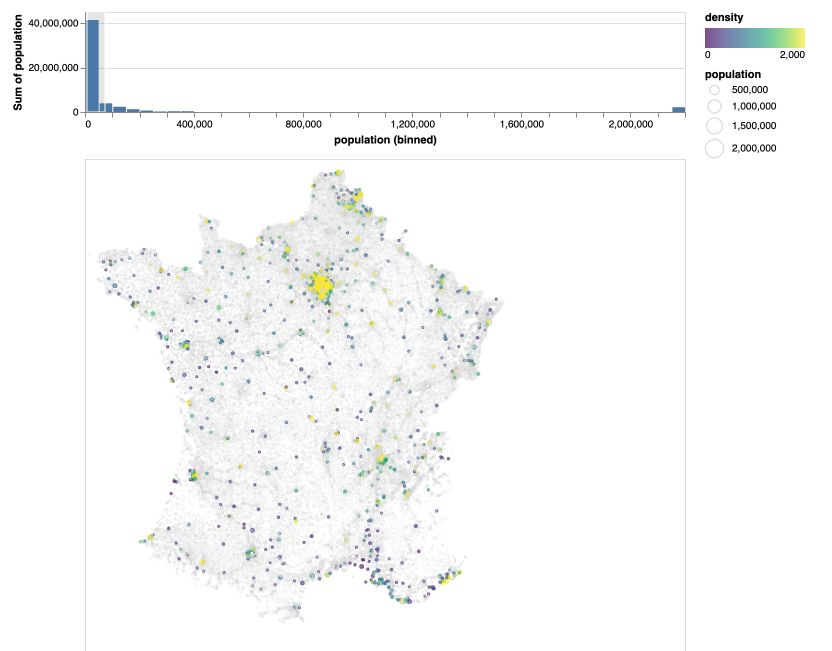Conclusion : That’s it ! I hope this introduction to Altair was interesting. I love to use this on my Data Viz projects. Feel free to leave a comment if you have one.

Categories:

Updated: